# Separable equations

## Separable differential equations

### Method of separation of variables

The method of separation of variables consists in all of the proper algebraic operations applied to a differential equation (either ordinary or partial) which allows to separate the terms in the equation depending to the variable they contain. In other words, this method allows "separable equations" to be rewritten in a way that all of the terms containing one of the variables present go to one side of the equal sign in the equation, while all of the terms pertaining to the other variable present go to the other side of the equal sign; and so, each side of the equation remains as a function described in terms of only one variable that can be integrated in order to solve for the variable itself.

As you may have noted already, in this case we are talking about differential equations containing only two variables (usually y as the dependent variable and x as the independent variable. Sometimes t is used instead of x). And so, separation of variables applied to an equation defined in terms of the two variables x and y, ends up being any algebraic operation (such as addition, subtraction, multiplication, division, roots, etc) which is applied to both sides of a mathematical equality so we can organize all of the terms of x in one side and all of the terms of y in the other side.

Therefore, there is no universal set of steps to follow while working through separation of variables calculus, which can even be referred to as algebra for this part, since even total differentials are taken as simple terms for practical purposes while separating. Consequently, while working through separation of variables examples it is up to you to decide which operations, and in what order should they be computed to the equation so you can set apart different variable terms.

For this lesson we will focus on solving separable differential equations as a method to find a particular solution for an ordinary differential equation. An equation is defined as separable if simple algebra operations can obtain a result such as the one discussed above (putting distinct variables in the equation apart in each side of the equality). Also, make sure you are familiarized with what a differential equation perse is before moving on through this lesson, if you do not have much experience with differential equations yet we recommend you to take a look at the introduction to differential equations lesson before you continue with our topic.

Before we continue onto the next section, let us say a few more words about the method of finding solutions to differential equations by separation of variables: The technique is one of the most used in order to solve first order separable differential equations, since is one of the simplest approaches we have to obtain their particular solutions, and even if you have problems to solve containing non separable differential equations, you can work through the first stages of the problem with different techniques (which you will see in later lessons) and at some point you may find out that you still will have to use separation after simplification has taken place.

In conclusion, separation of variables differential equations refer to those problems which contain a typical ordinary differential equation or a partial differential equation which is separable. Thus, the first thing you have to do to know if you can use this method or not while working on a given problem, is to know if you have a separable equation or not.

### How to tell if a differential equation is separable

Separable differential equations have the general form of: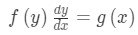Equation 1: General form of a separable differential equation
Where:
f(y) is a function in terms of y.
g(x) is a function in terms of x.
dy/dx is the rate of change of y in terms of x

In such equations you will find that a function of y multiplying the total derivative of y with respect to the independent variable (in this case x) will be equal to a function of x. In order to fully "separate" them, we need to have all x's in one side and so we move the term dx (total differential of x) to the right by "multiplying" it in both sides as if it was a common algebraic term: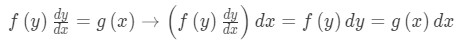Equation 2: Algebraic "trick" to separate a differential equation.

It is important to know this is a trick and not the true mathematical operation that goes on in this shift of dx from the left-hand side to the right-hand side. The way how the total differential dx moves involves a much complicated operation and comes from definition of total derivative in terms of partial derivatives. For practical purposes we will leave such topics for later and just follow this "trick" for the moment.

Notice that equation 1 will produce separable first order differential equations only, meaning that the highest derivative you will find in them is the first derivative of y. The reason for this is that we mostly use this technique on such equations since they tend to be the more manageable ones, and so, the ones we can rearrange to put the different variables apart.

We will talk about methods to solve equations containing higher order derivatives in future lessons, and although these will be much more mathematically robust, as we mentioned before, you will be happy to see that sometimes separation comes as an aid tool through some stages of higher level problems.

### How to solve separable differential equations

In order to solve separable differential equations you need to follow the next simple steps. For problems without initial values you need to find a general solution and thus arrive until step 3, for initial value problems (those with initial conditions) you have to go through all the steps in order to find a particular solution.

1. Put all of the y terms from the equation in one side and all of the x terms on the other.
2. Integrate each side.
1. For this step you may have to use different methods of integration depending on the equation you have to integrate. Such methods can be:
1. U-substitution
2. Integration by parts
3. Integration using trigonometric identities
4. Trigonometric substitution
5. Integration of rational functions by partial fractions
* Be sure to review these lessons so you are prepared for these antiderivatives.
3. Solve for y to obtain a general solution.
4. If an initial condition is given, apply the value to the general solution and find the value of the unknown constant c.
5. Obtain the particular solution by plugging the value of c into the general solution.

Remember these are the general steps to follow through the complete solution of a differential equation that is separable, but notice the separation part itself occurs only in step 1. As mentioned before, step one has no single universal solution and you have to figure out how to put all x's and y's apart.

Now that you have an easy set of instructions to follow, let us work through some separable differential equations examples:

### Example 1

Find the general solution to the following differential equation: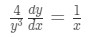Equation for example 1: Separable differential equation
• #### Step 1:

First we separate all of the y terms from the x terms by putting each type of term in a different side of the equal sign: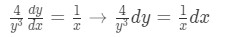Equation for example 1(a): Separating the terms of the differential equation
• #### Step 2:

We integrate each side, the side containing the y terms must be integrated with respect to y, and the side containing the x terms must be integrated with respect to x.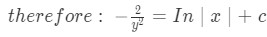Equation for example 1(b-1): Integrating both sides of the equation

Notice that in this case, we didn't put the constant value resulting from the left-hand side integration. Let us explain this explicitly: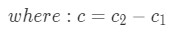Equation for example 1(b-2): Explicit explanation of the simplification of c

As you can see, if we pass this constant to the other side by subtracting it from both sides we will end up with a subtraction of unknown constants in the right-hand side, which at the end is just another unknown constant. This happens every time, and thus we have decided to just name this constant c and for practical purposes, always keep it in the side of the equation containing the terms of x.

• #### Step 3:

Now we solve for y in order to obtain our general solution: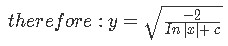Equation for example 1: (c-1): Solving for y

And so our general solution is: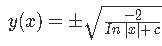Equation for example 1(c-2): General solution to the differential equation

Is important to note that the signs were added to our general solution equation because if we were to have an initial condition for the problem, the resultant value could be either positive or negative as long as their absolute value is the same. The reason for that is that it doesn't matter if a number is positive or negative, if you square it you will obtain a positive value, this positive value would be the one inside the square root in the equation above.
In simple words: all square roots have two possible solutions, a positive and a negative one where both have the same absolute value.

### Example 2

Find the general solution of the following differential equation: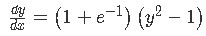Equation for example 2: Separable differential equation
• #### Step 1:

Separate all of the y terms from the x terms by putting them in different sides of the equal sign: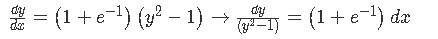Equation for example 2(a): Separating the terms of the differential equation
• #### Step 2:

Integrating each side: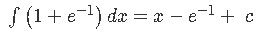Equation for example 2(b-1): Integrating both sides of the equation part 1

For the left hand-side of the equation we use the method of integration by partial fractions and the notable product called "difference of squares" → $\left(a^{\small2}-b^{\small2}\right)=\left(a+b\right)\left(a-b\right)$ so we can rewrite the left hand side as:

• In order to solve this integral, we first solve the fraction inside using partial fractions: plugging the value of B and setting y = 0 then: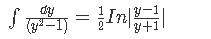Equation for example 2(b-2): Integrating both sides of the equation part 2
• #### Step 3:

Now we put both left hand-side and right hand-side together to obtain the general solution to the equation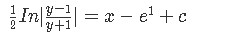Equation for example 2(c): General solution to the differential equation

In this case we will not be solving for y since this complicates the equation even more, therefore we just say this is the most practical way to represent the relationship between y and x and thus this is our general solution.

When solving for general solutions, always try to solve for y and simplify the expression as much as possible. For this problem, if we were to have an initial value condition we could apply it, solve for the unknown constant and then solve explicitly for y, probably simplifying the expression much more, but since no initial value was given, this is the most we can do for the moment.

### Example 3

Obtain the general solution for the differential equation below: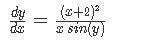Equation for example 3: Separable differential equation
• #### Step 1:

Separating the y and x terms: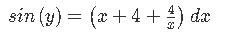Equation for example 3(a): Separating the terms of the differential equation
• #### Step 2:

Integrating each side of the equation:
therefore: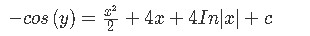Equation for example 3(b): Integrating both sides of the equation
• #### Step 3:

We solve for y in order to find the general solution for the differential equation. In this case we start by passing the negative sign to the right-hand side and then applying the inverse cosine to both sides to obtain the final result: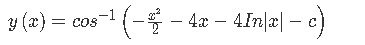Equation for example 3(c): General solution for the differential equation

### Example 4

Calculate the general solution for the differential equation below: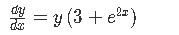Equation for example 4: Separable differential equation
• #### Step 1:

We separate the y and x terms: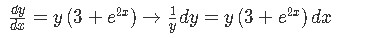Equation for example 4(a): Separating the terms of the differential equation
• #### Step 2:

Integrating each side of the equation:
therefore: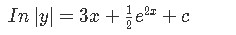Equation for example 4(b): Integrating both sides of the equation
• #### Step 3:

We apply the exponential to both sides to solve for y and find the general solution for the differential equation: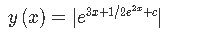Equation for example 4(c): General solution for the differential equation

### Example 5

Calculate the general solution for the differential equation below: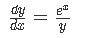Equation for example 5: Separable differential equation
• #### Step 1:

We separate the y and x terms: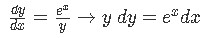Equation for example 5(a): Separating the terms of the differential equation
• #### Step 2:

Integrating each side of the equation:
therefore: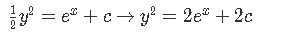Equation for example 5(b): Integrating both sides of the equation
• #### Step 3:

Having y squared we just take the square root of both sides of the equation in so we obtain the general solution for the differential equation: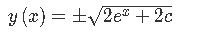Equation for example 5(c): General solution for the differential equation

Now is time for us to look into problems containing initial conditions, in other words, we will be solving differential equations for which you know one particular result. This way you can apply the information you know about this differential equation so you can find the value of the unknown constant and obtain the particular solution to the differential equation.

These problems are what we call, initial value problems, since they initially provide certain values in order to find a more specific solution. Remember that for these problems, we will go through the whole list of steps specified at the beginning of this section in order to find the solution.

### Example 6

Find the particular solution to the first order differential equation, use separation of variables to solve the initial value problem with condition y(0)=2.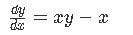Equation for example 6: Separable differential equation
• #### Step 1:

We separate the y and x terms: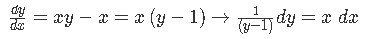Equation for example 6(a): Separating the terms of the differential equation
• #### Step 2:

Integrating each side of the equation:
therefore: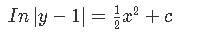Equation for example 6(b): Integrating both sides of the equation
• #### Step 3:

We apply the exponential to both sides and solve for y to find the general solution expression:
therefore: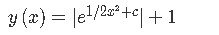Equation to example 6(c): General solution for the differential equation
• #### Step 4

Let us apply the initial condition y(0)=2 (which means that when x=0, y=2) in order to find the value of the unknown constant in the general solution.
therefore: c=0
• #### Step 5

And finally, we plug the value of c into the general solution to find the particular solution of the differential equation: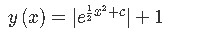Equation for example 6(f): Particular solution of the differential equation

Now let us take a look at our final example, also a problem in which you will find the particular solution:

### Example 7

Compute the particular solution for the differential equation below with initial condition y(0)=1.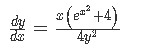Equation for example 7: Separable differential equation
• #### Step 1:

We separate the y and x terms: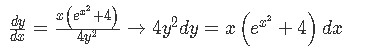Equation for example 7(a): Separating the terms of the differential equation
• #### Step 2:

Integrating each side of the equation:
therefore: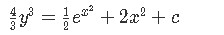Equation for example 7(b): Integrating both sides of the equation
• #### Step 3:

Now we solve for y to find the general solution:
therefore: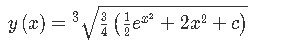Equation to example 7(c): General solution for the differential equation
• #### Step 4:

Almost done! We need to apply the initial condition y(0)=1 so we can find the value of c in the general solution.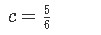Equation for example 7(d): Solving for the unknown constant from the general solution
• #### Step 5:

Finally, plugging the value of c into the general solution we obtain the particular solution for the differential equation: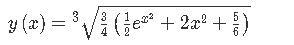Equation for example 7(f): Particular solution of the differential equation

We hope you have enjoyed these examples and that the technique to solve separable equations is clear now. This is an important lesson to understand completely since it will be used over and over again in later lessons. Make sure that you go through every single step of all of the examples a few times if you have issues.

As a last thing, we recommend you to take a look into these separable equations notes so you can see a few more examples to continue your studies.

### Separable equations

Separable of variables is a method we use to find a general solution of a differential equation. The method involves separating all the y variables to the left hand side of the equation, and moving all the x variables to the right side. Afterwards, we integrate both sides of the equation and then isolate for y to find the general solution. If it is too hard to isolate for y, you can leave the answer as it is. We will also be looking at questions about particular solutions. These are solutions which involves finding the value of the constant, when given an initial value.

#### Lessons

A separable differential equation is in the following form:

$f(y)\frac{dy}{dx}=g(x)$

Where:
1. $f(x)$ is a function in terms of $y$.
2. $g(x)$ is a function in terms of $x$.

We want to convert the equation to the following form:
$f(y)dy=g(x)dx$
so that we can integral both sides, and solve for $y$.

• Introduction
Separable Equations Overview

• 1.
Separable Equations without Initial Conditions
Find the general solution of the following differential equations:
a)
$\frac{4}{y^3}\frac{dy}{dx}=\frac{1}{x}$

b)
$\frac{dy}{dx}=(1+e^{-x})(y^2-1)$

c)
$\frac{dy}{dx}=\frac{(x+2)^2}{x \sin y}$

d)
$\frac{dy}{dx}=y(3+e^{2x})$

e)
$\frac{dy}{dx}=\frac{e^x}{y}$

• 2.
Initial Value Problems
Solve the following differential equations:
a)
$\frac{dy}{dx}=xy-x$ subject to $y(0)=2$

b)
$\frac{dy}{dx}=\frac{x(e^{x^2}+4)}{4y^2}$ subject to $y(0)=1$